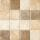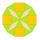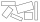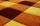# Area + divisibility - math problems

#### Number of problems found: 16

• TilesIt is given an area of 5m x 4m One tile is 40 x 40 cm How many tiles are needed in an area of 5 m x 4 m? And how many tiles need to be cut (if it not possible for the tiles to fall exactly)?
• CuboidThe volume of the cuboid is 245 cm3. Each cuboid edge length can be expressed by a integer greater than 1 cm. What is the surface area of the cuboid?
• Cuboid wallsCalculate the cuboid volume if its different walls have an area of 195cm², 135cm², and 117cm².
• Mr. ZucchiniMr. Zucchini had a rectangular garden whose perimeter is 28 meters. The garden's content area filled just four square beds, whose dimensions in meters are expressed in whole numbers. Determine what size could have a garden. Find all the possibilities and
• Gardens colonyGardens colony with dimensions of 180 m and 300 m are to be completely divided into the same large squares of the highest area. Calculate how many such squares can be obtained and determine the length of the square side.
• RectanglesHow many rectangles with area 8713 cm2 whose sides are natural numbers are?
• TilesThe tile has the shape of a square with a side of 15 cm. What dimensions can a rectangle composed of 90 of these tiles have so that no tile remains?
• TilesHall has dimensions 325 &time; 170 dm. What is the largest size of square tiles that can be entire hall tiled and how many we need them?
• TilesThe room has dimensions 12 m and 5.6 m. Determine the number of square tiles and their largest possible size to cover them room's floor.
• Square tilesThe room has dimensions of 12 meters and 5.6 meters. Determine the number of square tiles and their largest dimension to exactly cover the floor.
• Square roomWhat is the size of the smallest square room, which can pave with tiles with dimensions 55 cm and 45 cm? How many such tiles is needed?
• Paper squaresThe paper rectangle measuring 69 cm and 46 cm should be cut into as many squares as possible. Calculate the lengths of squares and their number.
• TilesFrom how many tiles 20 cm by 30 cm we can build a square of maximum dimensions, if we have maximum 881 tiles.
• PagansElena cut out same circle-shaped pagans and put them on a rectangular sheet so that the neighboring pagans were touching each other and the pagans were touching the walls of the sheet on the edges. Each pagans occupied 28.26 cm2 of the bottom of the sheet
• The carpetHow many meters of carpet 90 cm wide need to cover floor room which has a rectangular shape with a lengths 4.8 m and 2.4 m if the number of pieces on the carpet is needed to be lowest?
• PlumberThe plumber had to cut the metal strip with dimensions 380 cm and 60 cm to the largest squares to no waste. Calculate the length of the sides of a square. How many squares cut it?

Do you have an interesting mathematical word problem that you can't solve it? Submit a math problem, and we can try to solve it.

We will send a solution to your e-mail address. Solved examples are also published here. Please enter the e-mail correctly and check whether you don't have a full mailbox.

Please do not submit problems from current active competitions such as Mathematical Olympiad, correspondence seminars etc...

Area - math word problems. Divisibility - math word problems.